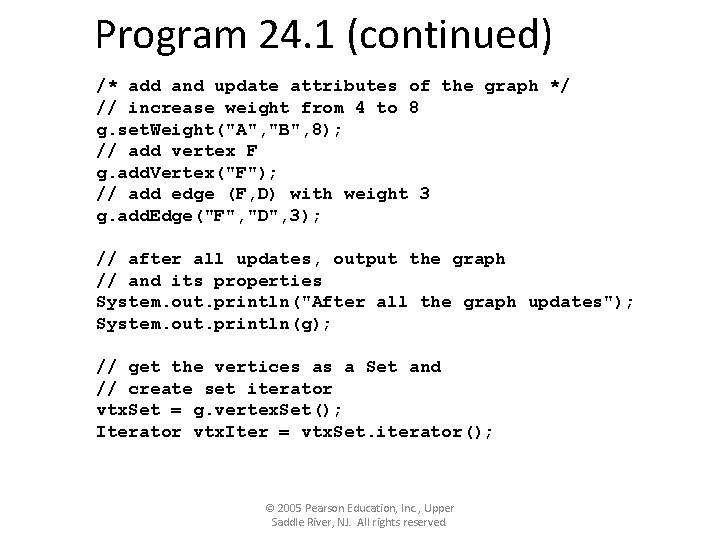# Graph Terminologi Graph A graph consists of a

• Slides: 29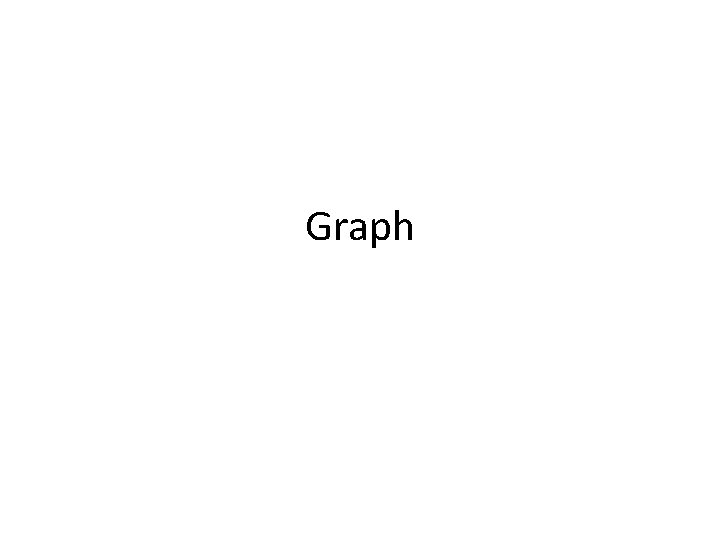Graph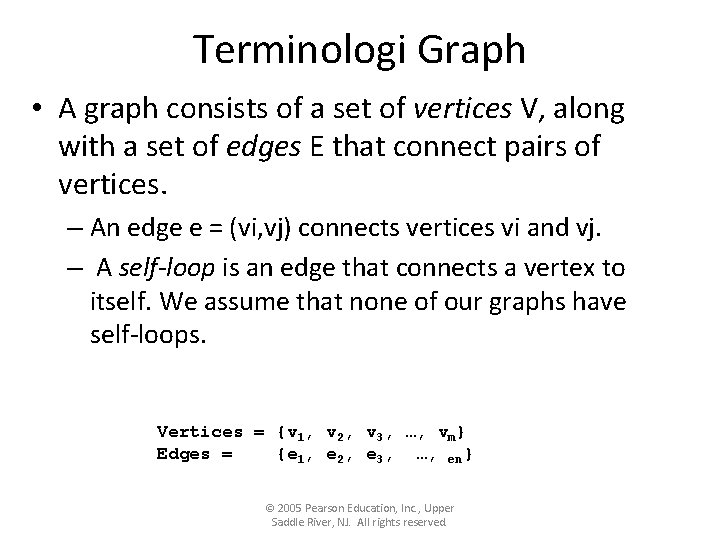Terminologi Graph • A graph consists of a set of vertices V, along with a set of edges E that connect pairs of vertices. – An edge e = (vi, vj) connects vertices vi and vj. – A self-loop is an edge that connects a vertex to itself. We assume that none of our graphs have self-loops. Vertices = {v 1, v 2, v 3, …, vm} Edges = {e 1, e 2, e 3, …, en} © 2005 Pearson Education, Inc. , Upper Saddle River, NJ. All rights reserved.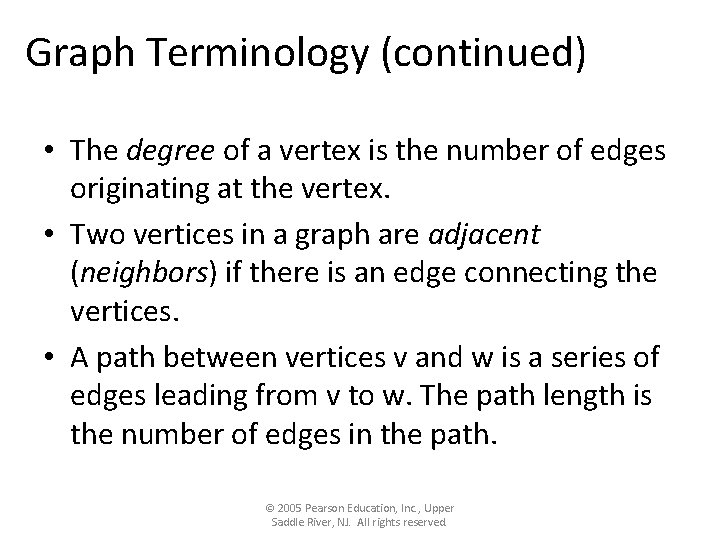Graph Terminology (continued) • The degree of a vertex is the number of edges originating at the vertex. • Two vertices in a graph are adjacent (neighbors) if there is an edge connecting the vertices. • A path between vertices v and w is a series of edges leading from v to w. The path length is the number of edges in the path. © 2005 Pearson Education, Inc. , Upper Saddle River, NJ. All rights reserved.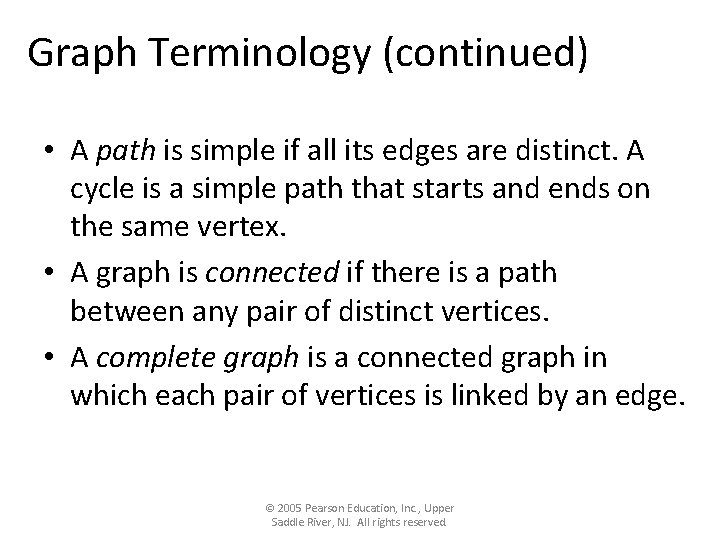Graph Terminology (continued) • A path is simple if all its edges are distinct. A cycle is a simple path that starts and ends on the same vertex. • A graph is connected if there is a path between any pair of distinct vertices. • A complete graph is a connected graph in which each pair of vertices is linked by an edge. © 2005 Pearson Education, Inc. , Upper Saddle River, NJ. All rights reserved.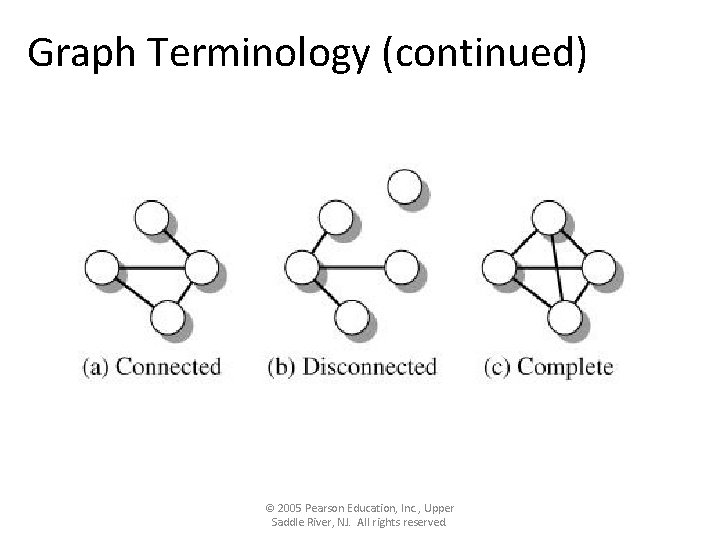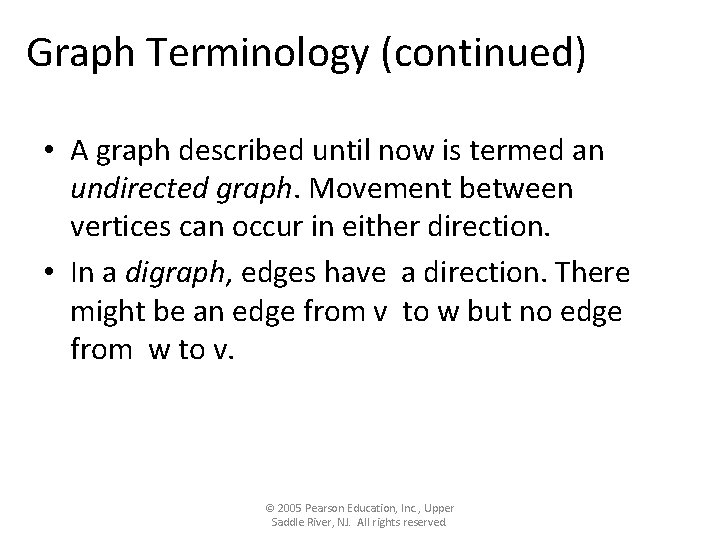Graph Terminology (continued) • A graph described until now is termed an undirected graph. Movement between vertices can occur in either direction. • In a digraph, edges have a direction. There might be an edge from v to w but no edge from w to v. © 2005 Pearson Education, Inc. , Upper Saddle River, NJ. All rights reserved.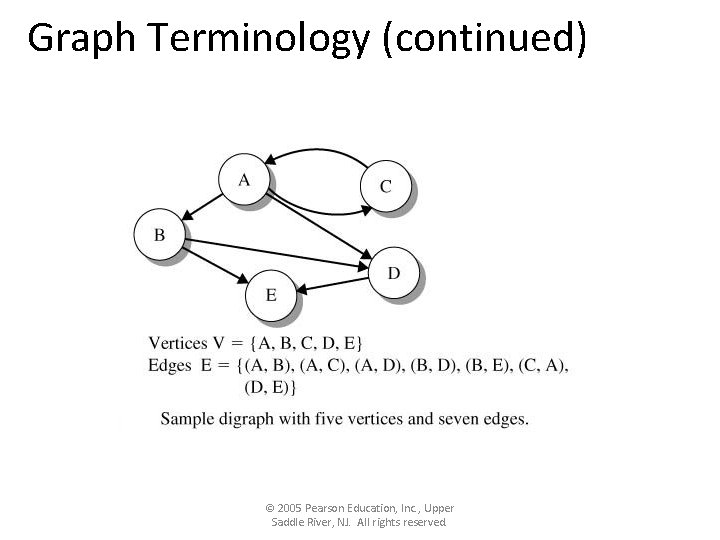Graph Terminology (continued) • In a digraph, a directed path (path) connecting vertices vs and ve is a sequence of directed edges that begin at vs and end at ve. • The number of the edges that emanate from a vertex v is called the out-degree of the vertex. • The number of the edges that terminate in vertex v is the in-degree of the vertex. © 2005 Pearson Education, Inc. , Upper Saddle River, NJ. All rights reserved.Graph Terminology (continued) • A digraph is strongly connected if there is a path from any vertex to any other vertex. • The digraph is weakly connected if, for each pair of vertices vi and vj, there is either a path P(vi, vj) or a path P(vj, vi). © 2005 Pearson Education, Inc. , Upper Saddle River, NJ. All rights reserved.Graph Terminology (concluded) • An acyclic graph has no cycles. • Each edge in a weighted digraph, has a cost associated with traversing the edge. © 2005 Pearson Education, Inc. , Upper Saddle River, NJ. All rights reserved.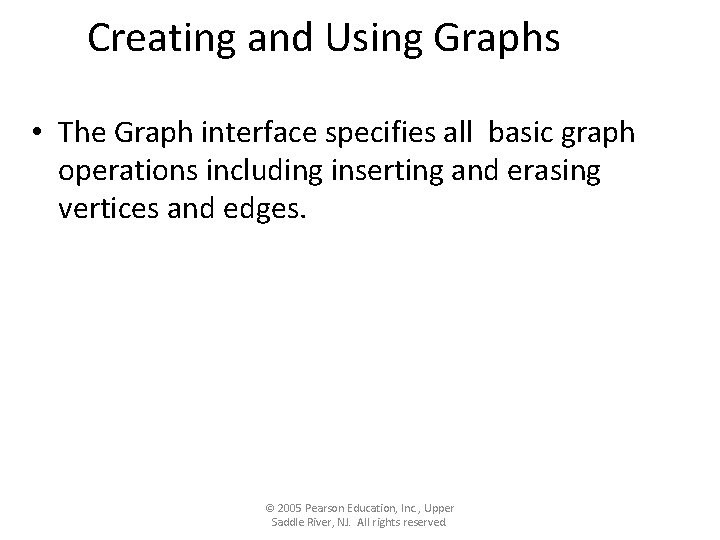Creating and Using Graphs • The Graph interface specifies all basic graph operations including inserting and erasing vertices and edges. © 2005 Pearson Education, Inc. , Upper Saddle River, NJ. All rights reserved.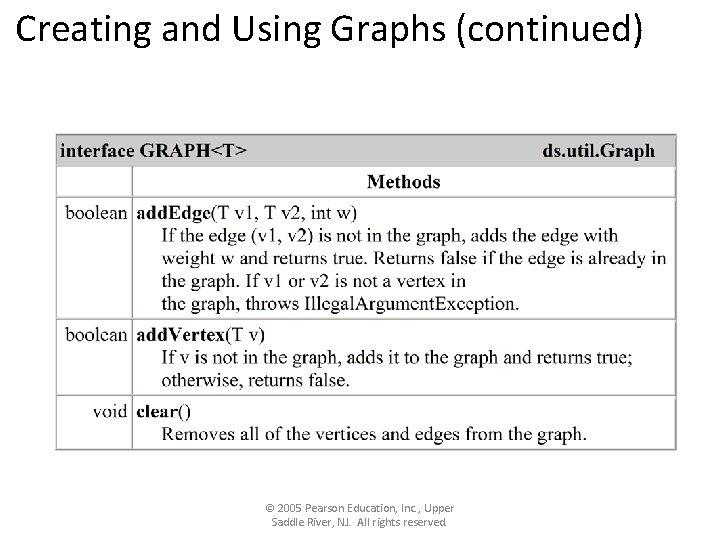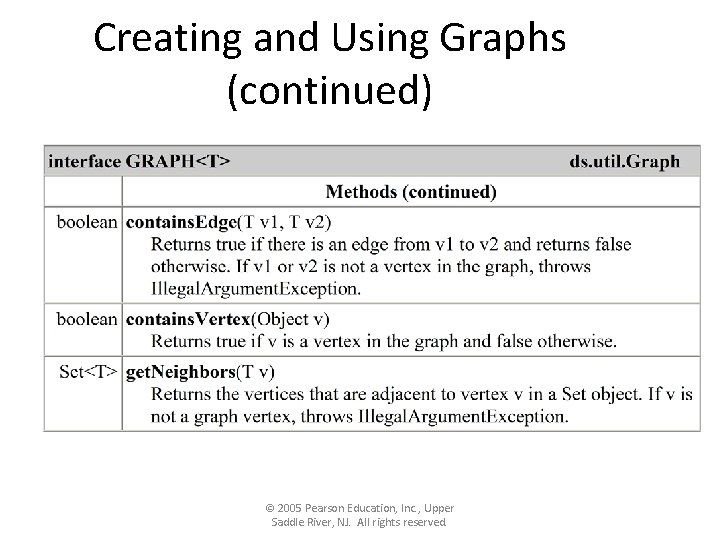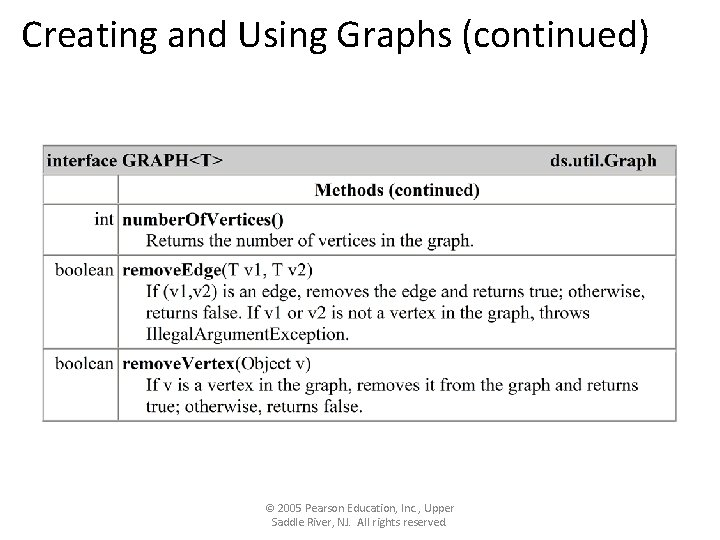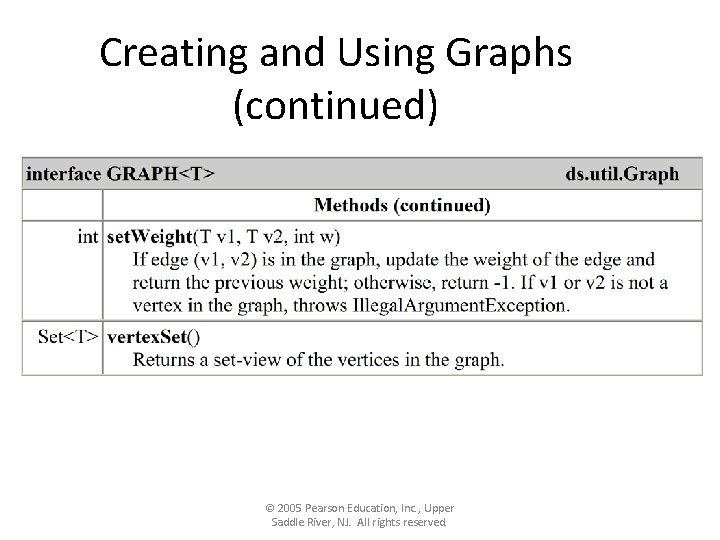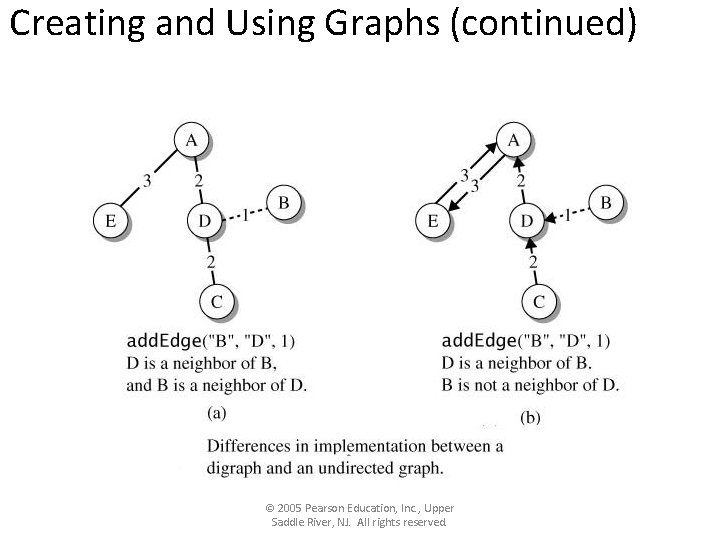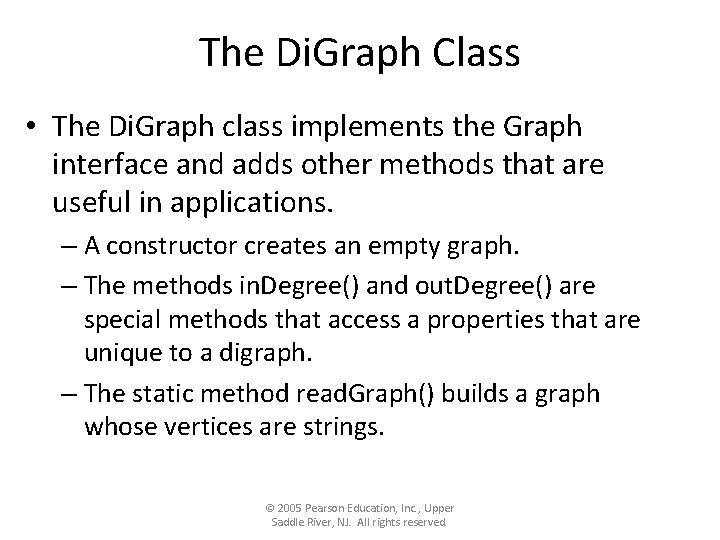The Di. Graph Class • The Di. Graph class implements the Graph interface and adds other methods that are useful in applications. – A constructor creates an empty graph. – The methods in. Degree() and out. Degree() are special methods that access a properties that are unique to a digraph. – The static method read. Graph() builds a graph whose vertices are strings. © 2005 Pearson Education, Inc. , Upper Saddle River, NJ. All rights reserved.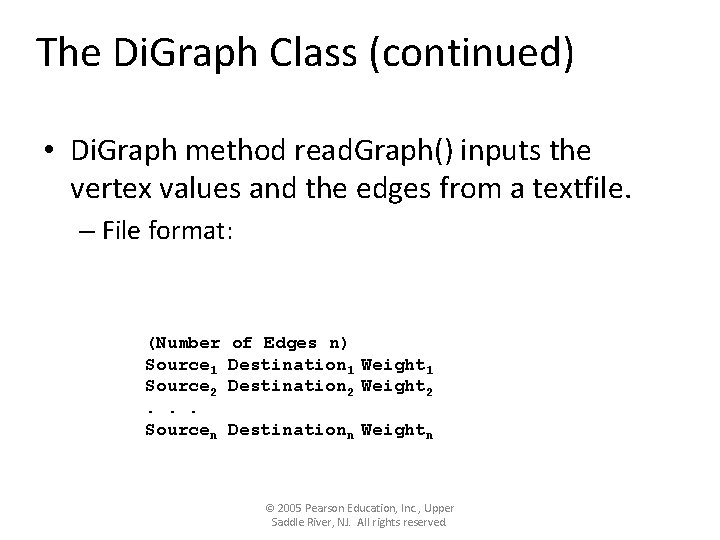The Di. Graph Class (continued) • Di. Graph method read. Graph() inputs the vertex values and the edges from a textfile. – File format: (Number Source 1 Source 2. . . Sourcen of Edges n) Destination 1 Weight 1 Destination 2 Weight 2 Destinationn Weightn © 2005 Pearson Education, Inc. , Upper Saddle River, NJ. All rights reserved.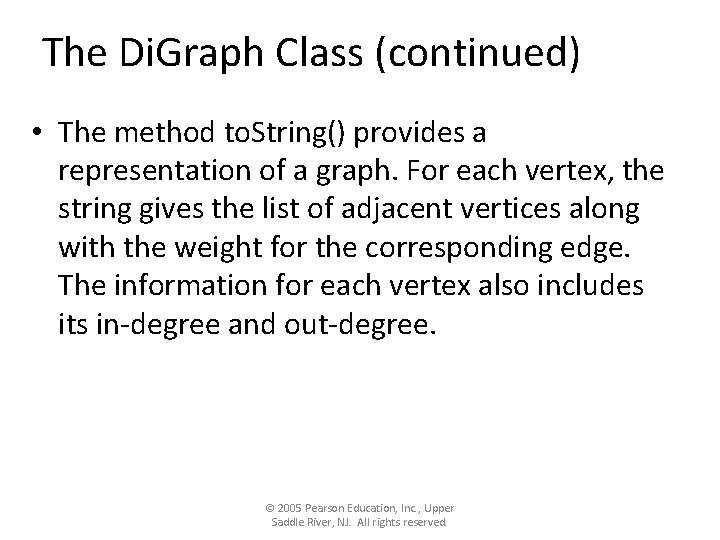The Di. Graph Class (continued) • The method to. String() provides a representation of a graph. For each vertex, the string gives the list of adjacent vertices along with the weight for the corresponding edge. The information for each vertex also includes its in-degree and out-degree. © 2005 Pearson Education, Inc. , Upper Saddle River, NJ. All rights reserved.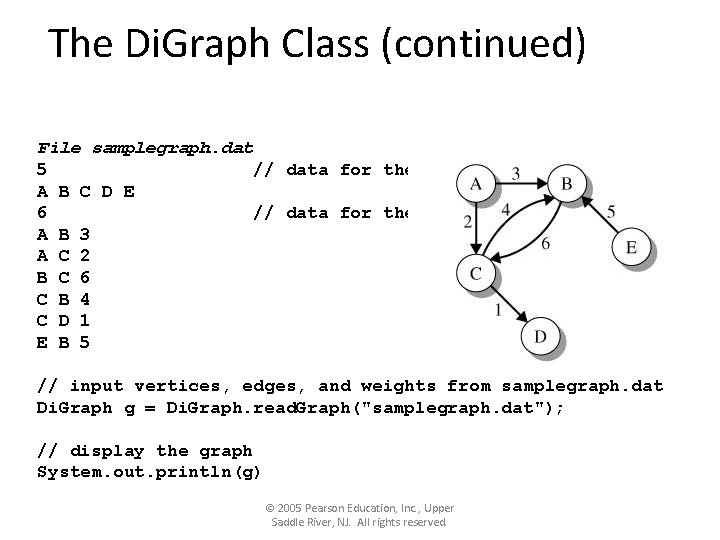The Di. Graph Class (continued) File samplegraph. dat 5 // data for the vertices A B C D E 6 // data for the edges A B 3 A C 2 B C 6 C B 4 C D 1 E B 5 // input vertices, edges, and weights from samplegraph. dat Di. Graph g = Di. Graph. read. Graph("samplegraph. dat"); // display the graph System. out. println(g) © 2005 Pearson Education, Inc. , Upper Saddle River, NJ. All rights reserved.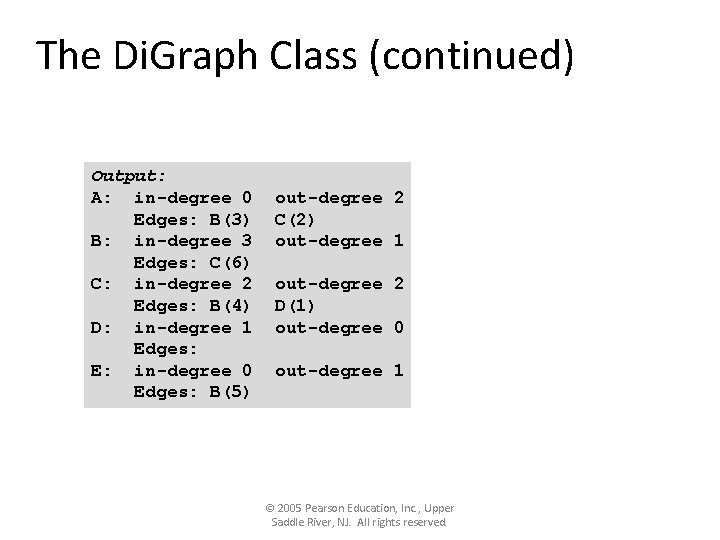The Di. Graph Class (continued) Output: A: in-degree 0 Edges: B(3) B: in-degree 3 Edges: C(6) C: in-degree 2 Edges: B(4) D: in-degree 1 Edges: E: in-degree 0 Edges: B(5) out-degree 2 C(2) out-degree 1 out-degree 2 D(1) out-degree 0 out-degree 1 © 2005 Pearson Education, Inc. , Upper Saddle River, NJ. All rights reserved.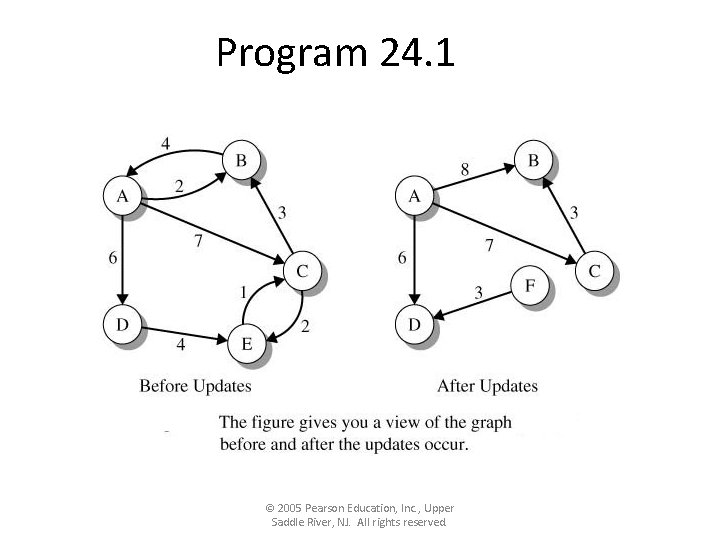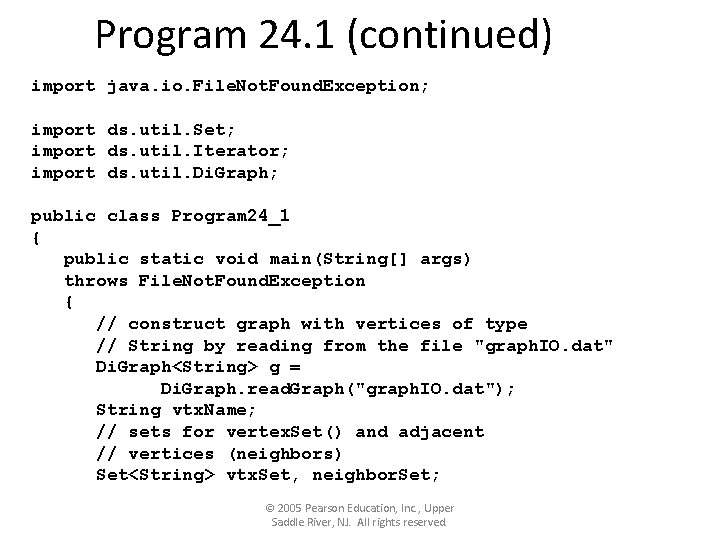Program 24. 1 (continued) import java. io. File. Not. Found. Exception; import ds. util. Set; import ds. util. Iterator; import ds. util. Di. Graph; public class Program 24_1 { public static void main(String[] args) throws File. Not. Found. Exception { // construct graph with vertices of type // String by reading from the file "graph. IO. dat" Di. Graph<String> g = Di. Graph. read. Graph("graph. IO. dat"); String vtx. Name; // sets for vertex. Set() and adjacent // vertices (neighbors) Set<String> vtx. Set, neighbor. Set; © 2005 Pearson Education, Inc. , Upper Saddle River, NJ. All rights reserved.Program 24. 1 (continued) // output number of vertices and edges System. out. println("Number of vertices: " + g. number. Of. Vertices()); System. out. println("Number of edges: " + g. number. Of. Edges()); // properties relative to vertex A System. out. println("in. Degree for A: " + g. in. Degree("A")); System. out. println("out. Degree for A: " + g. out. Degree("A")); System. out. println("Weight e(A, B): " + g. get. Weight("A", "B")); // delete edge with weight 2 g. remove. Edge("B", "A"); // delete vertex "E" and edges (E, C), // (C, E) and (D, E) g. remove. Vertex("E"); © 2005 Pearson Education, Inc. , Upper Saddle River, NJ. All rights reserved.